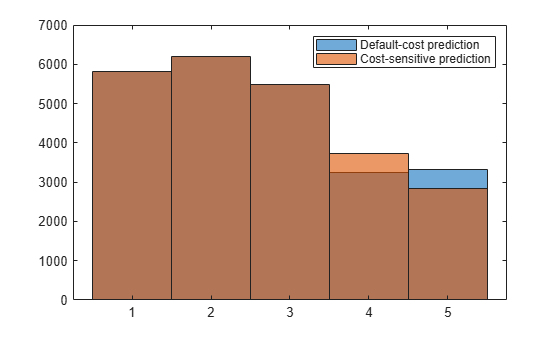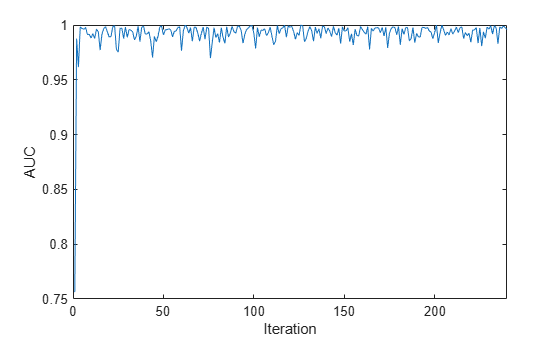# predict

Predict responses for new observations from naive Bayes incremental learning classification model

## Syntax

``label = predict(Mdl,X)``
``label = predict(Mdl,X,Name,Value)``
``[label,Posterior,Cost] = predict(___)``

## Description

example

````label = predict(Mdl,X)` returns the predicted responses (or labels) `label` of the observations in the predictor data `X` from the naive Bayes classification model for incremental learning `Mdl`.```

example

````label = predict(Mdl,X,Name,Value)` specifies additional options using one or more name-value arguments. For example, you can specify a custom misclassification cost matrix (in other words, override the value `Mdl.Cost`) for computing predictions by specifying the `Cost` argument.```

example

````[label,Posterior,Cost] = predict(___)` also returns the posterior probabilities (`Posterior`) and predicted (expected) misclassification costs (`Cost`) corresponding to the observations (rows) in `X` using any of the input argument combinations in the previous syntaxes. For each observation in `X`, the predicted class label corresponds to the minimum expected classification cost among all classes.```

## Examples

collapse all

Load the human activity data set.

`load humanactivity`

For details on the data set, enter `Description` at the command line.

Fit a naive Bayes classification model to the entire data set.

`TTMdl = fitcnb(feat,actid)`
```TTMdl = ClassificationNaiveBayes ResponseName: 'Y' CategoricalPredictors: [] ClassNames: [1 2 3 4 5] ScoreTransform: 'none' NumObservations: 24075 DistributionNames: {1x60 cell} DistributionParameters: {5x60 cell} Properties, Methods ```

`TTMdl` is a `ClassificationNaiveBayes` model object representing a traditionally trained model.

Convert the traditionally trained model to a naive Bayes classification model for incremental learning.

`IncrementalMdl = incrementalLearner(TTMdl)`
```IncrementalMdl = incrementalClassificationNaiveBayes IsWarm: 1 Metrics: [1x2 table] ClassNames: [1 2 3 4 5] ScoreTransform: 'none' DistributionNames: {1x60 cell} DistributionParameters: {5x60 cell} Properties, Methods ```

`IncrementalMdl` is an `incrementalClassificationNaiveBayes` model object prepared for incremental learning.

The `incrementalLearner` function initializes the incremental learner by passing learned conditional predictor distribution parameters to it, along with other information `TTMdl` learned from the training data. `IncrementalMdl` is warm (`IsWarm` is `1`), which means that incremental learning functions can start tracking performance metrics.

An incremental learner created from converting a traditionally trained model can generate predictions without further processing.

Predict class labels for all observations using both models.

```ttlabels = predict(TTMdl,feat); illables = predict(IncrementalMdl,feat); sameLabels = sum(ttlabels ~= illables) == 0```
```sameLabels = logical 1 ```

Both models predict the same labels for each observation.

This example shows how to apply misclassification costs for label prediction on incoming chunks of data, while maintaining a balanced misclassification cost for training.

Load the human activity data set. Randomly shuffle the data.

```load humanactivity n = numel(actid); rng(10); % For reproducibility idx = randsample(n,n); X = feat(idx,:); Y = actid(idx);```

Create a naive Bayes classification model for incremental learning. Specify the class names. Prepare the model for `predict` by fitting the model to the first 10 observations.

```Mdl = incrementalClassificationNaiveBayes(ClassNames=unique(Y)); initobs = 10; Mdl = fit(Mdl,X(1:initobs,:),Y(1:initobs)); canPredict = size(Mdl.DistributionParameters,1) == numel(Mdl.ClassNames)```
```canPredict = logical 1 ```

Consider severely penalizing the model for misclassifying "running" (class 4). Create a cost matrix that applies 100 times the penalty for misclassifying running as compared to misclassifying any other class. Rows correspond to the true class, and columns correspond to the predicted class.

```k = numel(Mdl.ClassNames); Cost = ones(k) - eye(k); Cost(4,:) = Cost(4,:)*100; % Penalty for misclassifying "running" Cost```
```Cost = 5×5 0 1 1 1 1 1 0 1 1 1 1 1 0 1 1 100 100 100 0 100 1 1 1 1 0 ```

Simulate a data stream, and perform the following actions on each incoming chunk of 100 observations.

1. Call `predict` to predict labels for each observation in the incoming chunk of data.

2. Call `predict` again, but specify the misclassification costs by using the `Cost` argument.

3. Call `fit` to fit the model to the incoming chunk. Overwrite the previous incremental model with a new one fitted to the incoming observations.

```numObsPerChunk = 100; nchunk = ceil((n - initobs)/numObsPerChunk); labels = zeros(n,1); cslabels = zeros(n,1); cst = zeros(n,5); cscst = zeros(n,5); % Incremental learning for j = 1:nchunk ibegin = min(n,numObsPerChunk*(j-1) + 1 + initobs); iend = min(n,numObsPerChunk*j + initobs); idx = ibegin:iend; [labels(idx),~,cst(idx,:)] = predict(Mdl,X(idx,:)); [cslabels(idx),~,cscst(idx,:)] = predict(Mdl,X(idx,:),Cost=Cost); Mdl = fit(Mdl,X(idx,:),Y(idx)); end labels = labels((initobs + 1):end); cslabels = cslabels((initobs + 1):end);```

Compare the predicted class distributions between the prediction methods by plotting histograms.

```figure; histogram(labels); hold on histogram(cslabels); legend(["Default-cost prediction" "Cost-sensitive prediction"])```Because the cost-sensitive prediction method penalizes misclassifying class 4 so severely, more predictions into class 4 result as compared to the prediction method that uses the default, balanced cost.

Load the human activity data set. Randomly shuffle the data.

```load humanactivity n = numel(actid); rng(10) % For reproducibility idx = randsample(n,n); X = feat(idx,:); Y = actid(idx);```

For details on the data set, enter `Description` at the command line.

Create a naive Bayes classification model for incremental learning. Specify the class names. Prepare the model for `predict` by fitting the model to the first 10 observations.

```Mdl = incrementalClassificationNaiveBayes('ClassNames',unique(Y)); initobs = 10; Mdl = fit(Mdl,X(1:initobs,:),Y(1:initobs)); canPredict = size(Mdl.DistributionParameters,1) == numel(Mdl.ClassNames)```
```canPredict = logical 1 ```

`Mdl` is an `incrementalClassificationNaiveBayes` model. All its properties are read-only. The model is configured to generate predictions.

Simulate a data stream, and perform the following actions on each incoming chunk of 100 observations.

1. Call `predict` to compute class posterior probabilities for each observation in the incoming chunk of data.

2. Call `rocmetrics` to compute the area under the ROC curve (AUC) using the class posterior probabilities, and store the AUC value, averaged over all classes. This AUC is an incremental measure of how well the model predicts the activities on average.

3. Call `fit` to fit the model to the incoming chunk. Overwrite the previous incremental model with a new one fitted to the incoming observations.

```numObsPerChunk = 100; nchunk = floor((n - initobs)/numObsPerChunk); auc = zeros(nchunk,1); classauc = 5; % Incremental learning for j = 1:nchunk ibegin = min(n,numObsPerChunk*(j-1) + 1 + initobs); iend = min(n,numObsPerChunk*j + initobs); idx = ibegin:iend; [~,posterior] = predict(Mdl,X(idx,:)); mdlROC = rocmetrics(Y(idx),posterior,Mdl.ClassNames); [~,~,~,auc(j)] = average(mdlROC,'micro'); Mdl = fit(Mdl,X(idx,:),Y(idx)); end```

Now, `Mdl` is an `incrementalClassificationNaiveBayes` model object trained on all the data in the stream.

Plot the AUC values for each incoming chunk of data.

```plot(auc) xlim([0 nchunk]) ylabel('AUC') xlabel('Iteration')```The plot suggests that the classifier predicts the activities well during incremental learning.

## Input Arguments

collapse all

Naive Bayes classification model for incremental learning, specified as an `incrementalClassificationNaiveBayes` model object. You can create `Mdl` directly or by converting a supported, traditionally trained machine learning model using the `incrementalLearner` function. For more details, see the corresponding reference page.

You must configure `Mdl` to predict labels for a batch of observations.

• If `Mdl` is a converted, traditionally trained model, you can predict labels without any modifications.

• Otherwise, `Mdl.DistributionParameters` must be a cell matrix with `Mdl.NumPredictors` > 0 columns and at least one row, where each row corresponds to each class name in `Mdl.ClassNames`.

Batch of predictor data for which to predict labels, specified as an n-by-`Mdl.NumPredictors` floating-point matrix.

Data Types: `single` | `double`

### Name-Value Arguments

Specify optional pairs of arguments as `Name1=Value1,...,NameN=ValueN`, where `Name` is the argument name and `Value` is the corresponding value. Name-value arguments must appear after other arguments, but the order of the pairs does not matter.

Example: `Cost=[0 2;1 0]` attributes double the penalty for misclassifying observations with true class `Mdl.ClassNames(1)`, than for misclassifying observations with true class `Mdl.ClassNames(2)`.

Cost of misclassifying an observation, specified as a value in the table, where c is the number of classes in `Mdl.ClassNames`. The specified value overrides the value of `Mdl.Cost`.

ValueDescription
c-by-c numeric matrix

`Cost(i,j)` is the cost of classifying an observation into class `j` when its true class is `i`, for classes `Mdl.ClassNames(i)` and `Mdl.ClassNames(j)`. In other words, the rows correspond to the true class and the columns correspond to the predicted class. For example, `Cost = [0 2;1 0]` applies double the penalty for misclassifying `Mdl.ClassNames(1)` than for misclassifying `Mdl.ClassNames(2)`.

Structure array

A structure array having two fields:

• `ClassNames` containing the class names, the same value as `Mdl.ClassNames`

• `ClassificationCosts` containing the cost matrix, as previously described.

Example: `Cost=struct('ClassNames',Mdl.ClassNames,'ClassificationCosts',[0 2; 1 0])`

Data Types: `single` | `double` | `struct`

Prior class probabilities, specified as a value in this numeric vector. `Prior` has the same length as the number of classes in `Mdl.ClassNames`, and the order of the elements corresponds to the class order in `Mdl.ClassNames`. `predict` normalizes the vector so that the sum of the result is 1.

The specified value overrides the value of `Mdl.Prior`.

Data Types: `single` | `double`

Score transformation function describing how incremental learning functions transform raw response values, specified as a character vector, string scalar, or function handle. The specified value overrides the value of `Mdl.ScoreTransform`.

This table describes the available built-in functions for score transformation.

ValueDescription
`"doublelogit"`1/(1 + e–2x)
`"invlogit"`log(x / (1 – x))
`"ismax"`Sets the score for the class with the largest score to 1, and sets the scores for all other classes to 0
`"logit"`1/(1 + ex)
`"none"` or `"identity"`x (no transformation)
`"sign"`–1 for x < 0
0 for x = 0
1 for x > 0
`"symmetric"`2x – 1
`"symmetricismax"`Sets the score for the class with the largest score to 1, and sets the scores for all other classes to –1
`"symmetriclogit"`2/(1 + ex) – 1

Data Types: `char` | `string`

## Output Arguments

collapse all

Predicted responses (labels), returned as a categorical or character array; floating-point, logical, or string vector; or cell array of character vectors with n rows. n is the number of observations in `X`, and `label(j)` is the predicted response for observation `j`.

`label` has the same data type as the class names stored in `Mdl.ClassNames`. (The software treats string arrays as cell arrays of character vectors.)

Class posterior probabilities, returned as an n-by-`numel(Mdl.ClassNames)` floating-point matrix. `Posterior(j,k)` is the posterior probability that observation `j` is in class `k`. `Mdl.ClassNames` specifies the order of the classes.

Expected misclassification costs, returned as an n-by-`numel(Mdl.ClassNames)` floating-point matrix.

`Cost(j,k)` is the expected cost of the observation in row `j` of `X` being classified into class `k` (`Mdl.ClassNames(k)`).

collapse all

### Misclassification Cost

A misclassification cost is the relative severity of a classifier labeling an observation into the wrong class.

Two types of misclassification cost exist: true and expected. Let K be the number of classes.

• True misclassification cost — A K-by-K matrix, where element (i,j) indicates the cost of classifying an observation into class j if its true class is i. The software stores the misclassification cost in the property `Mdl.Cost`, and uses it in computations. By default, `Mdl.Cost(i,j)` = 1 if `i``j`, and `Mdl.Cost(i,j)` = 0 if `i` = `j`. In other words, the cost is `0` for correct classification and `1` for any incorrect classification.

• Expected misclassification cost — A K-dimensional vector, where element k is the weighted average cost of classifying an observation into class k, weighted by the class posterior probabilities.

`${c}_{k}=\sum _{j=1}^{K}\stackrel{^}{P}\left(Y=j|{x}_{1},...,{x}_{P}\right)Cos{t}_{jk}.$`

In other words, the software classifies observations into the class with the lowest expected misclassification cost.

### Posterior Probability

The posterior probability is the probability that an observation belongs in a particular class, given the data.

For naive Bayes, the posterior probability that a classification is k for a given observation (x1,...,xP) is

`$\stackrel{^}{P}\left(Y=k|{x}_{1},..,{x}_{P}\right)=\frac{P\left({X}_{1},...,{X}_{P}|y=k\right)\pi \left(Y=k\right)}{P\left({X}_{1},...,{X}_{P}\right)},$`

where:

• $P\left({X}_{1},...,{X}_{P}|y=k\right)$ is the conditional joint density of the predictors given they are in class k. `Mdl.DistributionNames` stores the distribution names of the predictors.

• π(Y = k) is the class prior probability distribution. `Mdl.Prior` stores the prior distribution.

• $P\left({X}_{1},..,{X}_{P}\right)$ is the joint density of the predictors. The classes are discrete, so $P\left({X}_{1},...,{X}_{P}\right)=\sum _{k=1}^{K}P\left({X}_{1},...,{X}_{P}|y=k\right)\pi \left(Y=k\right).$

## Version History

Introduced in R2021a